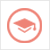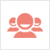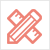﻿ 更多范文-Assignment代写：sql questions-51Due留学教育

#### 服务承诺资金托管原创保证实力保障24小时客服使命必达

#### 关于我们

51Due提供Essay，Paper，Report，Assignment等学科作业的代写与辅导，同时涵盖Personal Statement，转学申请等留学文书代写。51Due将让你达成学业目标51Due将让你达成学业目标51Due将让你达成学业目标51Due将让你达成学业目标

#### 名企实习积累工作经验多元化文化交流专业实操技能建立人际资源圈

# Assignment代写：sql questions

2018-04-11 来源: 51due教员组 类别: 更多范文Question 1 (5 marks)

Consider two relations A and B. A is of size 10,000 disk pages, and B is of size 1,000 pages. Consider the following SQL statement:

SELECT *

FROM A, B

WHERE A.a = B.a;

We wish to evaluate an equijoin between A and B, with an equality condition A.a = B.a. There are 502 buffer pages available for this operation. Both relations are stored as simple heap files. Neither relation has any indexes built on it.

Consider alternative join strategies described below and calculate the cost of each alternative. Evaluate the algorithms using the number of disk I/O’s as the cost. For each strategy, provide the formulae you use to calculate your cost estimates.

a) Page-oriented Nested Loops Join. Consider A as the outer relation. (1 mark)

b) Block-oriented Nested Loops Join. Consider A as the outer relation. (1 mark)

c) Sort-Merge Join (1 mark)

d) Hash Join (1 mark)

e) What would the lowest possible I/O cost be for joining A and B using any join algorithm and how much buffer space would be needed to achieve this cost? Explain briefly. (1 mark)

Question 2 (5 marks)

Consider a relation with the following schema:

Executives (id: integer, name:string, title:string, level: integer)

The Executives relation consists of 100,000 tuples stored in disk pages. The relation is stored as simple heap file and each page stores 100 tuples. There are 10 distinct titles in the Executives hierarchy and 20 distinct levels ranging from 0-20.

1

Suppose that the following SQL query is executed frequently using the given relation:

SELECT E.ename

FROM Executives

WHERE E.title = “CEO” and E.level > 15;

Your job is to analyze the query plans given below and estimate the cost of the best plan utilizing the information given about different indexes in each part.

a) Compute the estimated result size and the reduction factor (selectivity) of this query (1 mark)

b) Compute the estimated cost of the best plan assuming that a clustered B+ tree index on (title, level) is (the only index) available. Suppose there are 200 index pages, and the index uses Alternative 2. Discuss and calculate alternative plans. (1 mark)

c) Compute the estimated cost of the best plan assuming that an unclustered B+ tree index on (level) is (the only index) available. Suppose there are 200 index pages, and the index uses Alternative 2. Discuss and calculate alternative plans. (1 mark)

d) Compute the estimated cost of the best plan assuming that an unclustered Hash index on (title) is (the only index) available. The index uses Alternative 2. Discuss and calculate alternative plans. (1 mark)

e) Compute the estimated cost of the best plan assuming that an unclustered Hash index on (level) is (the only index) available. The index uses Alternative 2. Discuss and calculate alternative plans. (1 mark)

Question 3 (10 marks)

Consider the following relational schema and SQL query. The schema captures information about employees, departments, and company finances (organized on a per department basis).

Emp(eid: integer, did: integer, sal: integer, hobby: char(20)) Dept(did: integer, dname: char(20), floor: integer, phone: char(10)) Finance(did: integer, budget: real, sales: real, expenses: real)

Consider the following query:

SELECT D.dname, F.budget

FROM Emp E, Dept D, Finance F

WHERE E.did=D.did AND D.did=F.did

AND E.sal ≥ 59000 AND E.hobby = ‘yodeling’; 2

The system’s statistics indicate that employee salaries range from 10,000 to 60,000, and employees enjoy 200 different hobbies. There are a total of 50,000 employees and 5,000 departments (each with corresponding financial record in the Finance relation) in the database. Each relation fits 100 tuples in a page. Suppose there exists a clustered B+ tree index on (Emp.did) of size 50 pages.

a) Compute the estimated result size and the reduction factors (selectivity) of this query

(2 marks)

b) Compute the cost of the plans shown below. Assume that sorting of any relation (if required) can be done in 2 passes: 1st pass to produce sorted runs and 2nd pass to merge runs. Similarly hash join can be done in 2 passes: 1st pass to produce partitions, 2nd pass to join corresponding partitions. NLJ is a Page-oriented Nested Loops Join. Assume that did is the candidate key, and that 100 tuples of a resulting join between Emp and Dept fit in a page. Similarly, 100 tuples of a resulting join between Finance and Dept fit in a page. (8 marks, 2 marks per plan)

3

Formatting Requirements

For each question, present an answer in the following format:

Show the question number and question in black text